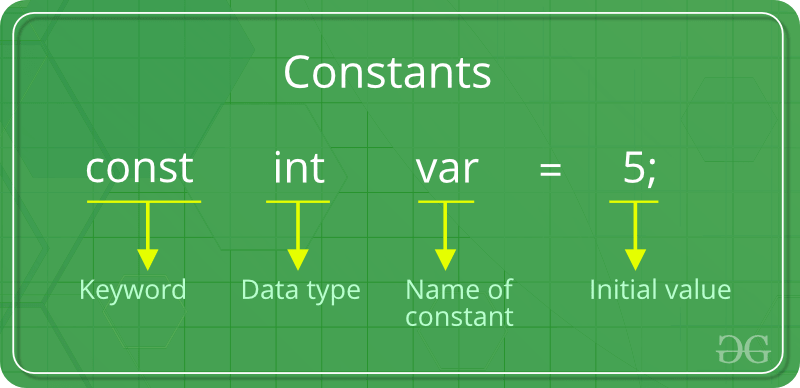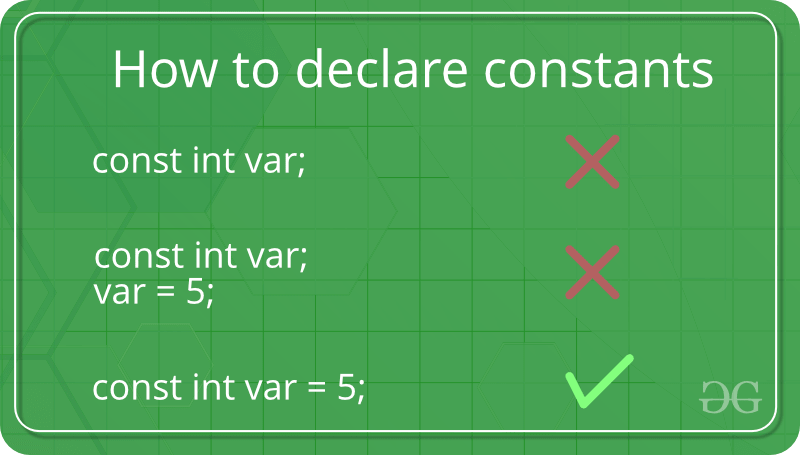# Constants in C

As the name suggests the name constants is given to such variables or values in C/C++ programming language which cannot be modified once they are defined. They are fixed values in a program. There can be any types of constants like integer, float, octal, hexadecimal, character constants etc. Every constant has some range. The integers that are too big to fit into an int will be taken as long. Now there are various ranges that differ from unsigned to signed bits. Under the signed bit, the range of an int varies from -128 to +127 and under the unsigned bit, int varies from 0 to 255.Defining Constants:
In C/C++ program we can define constants in two ways as shown below:

1. Using #define preprocessor directive
2. Using a const keyword

Literals: The values assigned to each constant variables are referred to as the literals. Generally, both terms, constants and literals are used interchangeably. For eg, “const int = 5;“, is a constant expression and the value 5 is refered to as constant integer literal.

Let us now learn about above two ways in details:

1. Using #define preprocessor directive: This directive is used to declare an alias name for existing variable or any value. We can use this to declare a constant as shown below:

`#define identifierName value`
• identifierName: It is the name given to constant.
• value: This refers to any value assigned to identifierName.

Example:

## C

 `#include  ` `#define val 10  ` `#define floatVal 4.5  ` `#define charVal 'G'  ` ` `  `int` `main()  ` `{  ` `    ``printf``(``"Integer Constant: %d\n"``,val);  ` `    ``printf``(``"Floating point Constant: %.1f\n"``,floatVal);  ` `    ``printf``(``"Character Constant: %c\n"``,charVal);  ` `     `  `    ``return` `0;  ` `}  `

## C++

 `#include ` `using` `namespace` `std; ` ` `  `#define val 10  ` `#define floatVal 4.5  ` `#define charVal 'G'  ` ` `  `int` `main() { ` `    ``cout << ``"Integer Constant: "` `<< val << ``"\n"``;  ` `    ``cout << ``"Floating point Constant: "` `<< floatVal << ``"\n"``;  ` `    ``cout << ``"Character Constant: "``<< charVal << ``"\n"``;  ` `     `  `    ``return` `0;  ` `} `

Output:

```Integer Constant: 10
Floating point Constant: 4.5
Character Constant: G
```

Refer Macros and Preprocessors in C for details.

2. using a const keyword: Using const keyword to define constants is as simple as defining variables, the difference is you will have to precede the definition with a const keyword.Below program shows how to use const to declare costants of different data types:

## C

 `#include ` ` `  `int` `main() ` `{ ` `    ``// int constant ` `    ``const` `int` `intVal = 10;  ` ` `  `    ``// Real constant ` `    ``const` `float` `floatVal = 4.14; ` `  `  `    ``// char constant  ` `    ``const` `char` `charVal = ``'A'``;  ` ` `  `    ``// string constant ` `    ``const` `char` `stringVal = ``"ABC"``;  ` `     `  `    ``printf``(``"Integer constant:%d \n"``, intVal ); ` `    ``printf``(``"Floating point constant: %.2f\n"``, floatVal ); ` `    ``printf``(``"Character constant: %c\n"``, charVal ); ` `    ``printf``(``"String constant: %s\n"``, stringVal); ` `     `  `    ``return` `0; ` `} `

## C++

 `#include ` `using` `namespace` `std; ` `  `  `int` `main() { ` `    ``// int constant ` `    ``const` `int` `intVal = 10;  ` ` `  `    ``// Real constant ` `    ``const` `float` `floatVal = 4.14; ` `  `  `    ``// char constant  ` `    ``const` `char` `charVal = ``'A'``;  ` ` `  `    ``// string constant ` `    ``const` `string stringVal = ``"ABC"``;  ` `      `  `    ``cout << ``"Integer Constant: "` `<< intVal << ``"\n"``;  ` `    ``cout << ``"Floating point Constant: "` `<< floatVal << ``"\n"``;  ` `    ``cout << ``"Character Constant: "``<< charVal << ``"\n"``;  ` `    ``cout << ``"String Constant: "``<< stringVal << ``"\n"``; ` `      `  `    ``return` `0;  ` `} `

Output:

```Integer constant: 10
Floating point constant: 4.14
Character constant: A
String constant: ABC
```

This article is contributed by Chinmoy Lenka. If you like GeeksforGeeks and would like to contribute, you can also write an article using contribute.geeksforgeeks.org or mail your article to contribute@geeksforgeeks.org. See your article appearing on the GeeksforGeeks main page and help other Geeks.

Please write comments if you find anything incorrect, or you want to share more information about the topic discussed above.

My Personal Notes arrow_drop_up

Article Tags :
Practice Tags :

5

Please write to us at contribute@geeksforgeeks.org to report any issue with the above content.